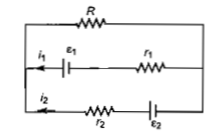NEET Physics Current Electricity Questions Solved

See the electrical circuit shown in this figure. Which of the following equation is a correct equation for it?(a) ${\epsilon }_{1}-\left({i}_{1}+{i}_{2}\right)R-{i}_{1}{r}_{1}=0$

(b) ${\epsilon }_{2}-{i}_{2}{r}_{2}-{\epsilon }_{1}-{i}_{1}{r}_{1}=0$

(c) $-{\epsilon }_{2}-\left({i}_{1}+{i}_{2}\right)R+{i}_{2}{r}_{2}=0$

(d) ${\epsilon }_{1}-\left({i}_{1}+{i}_{2}\right)R+{i}_{1}{r}_{1}=0$

Explanation is a part of a Paid Course. To view Explanation Please buy the course.

Difficulty Level: